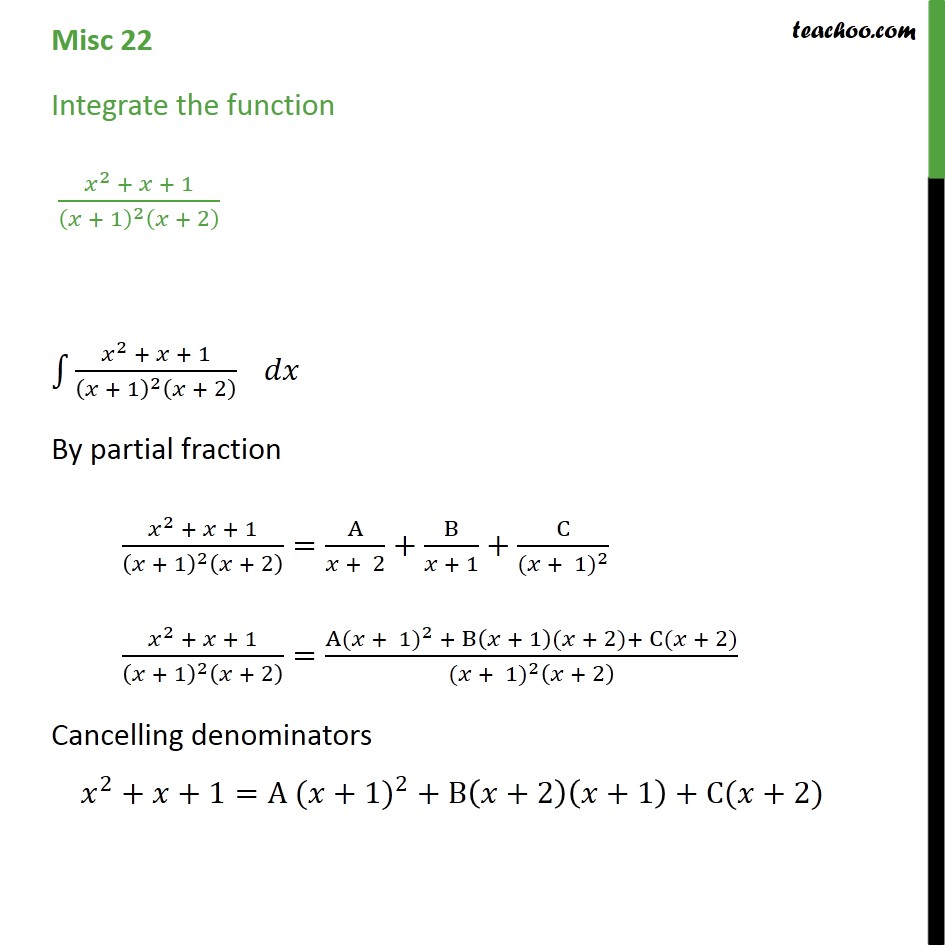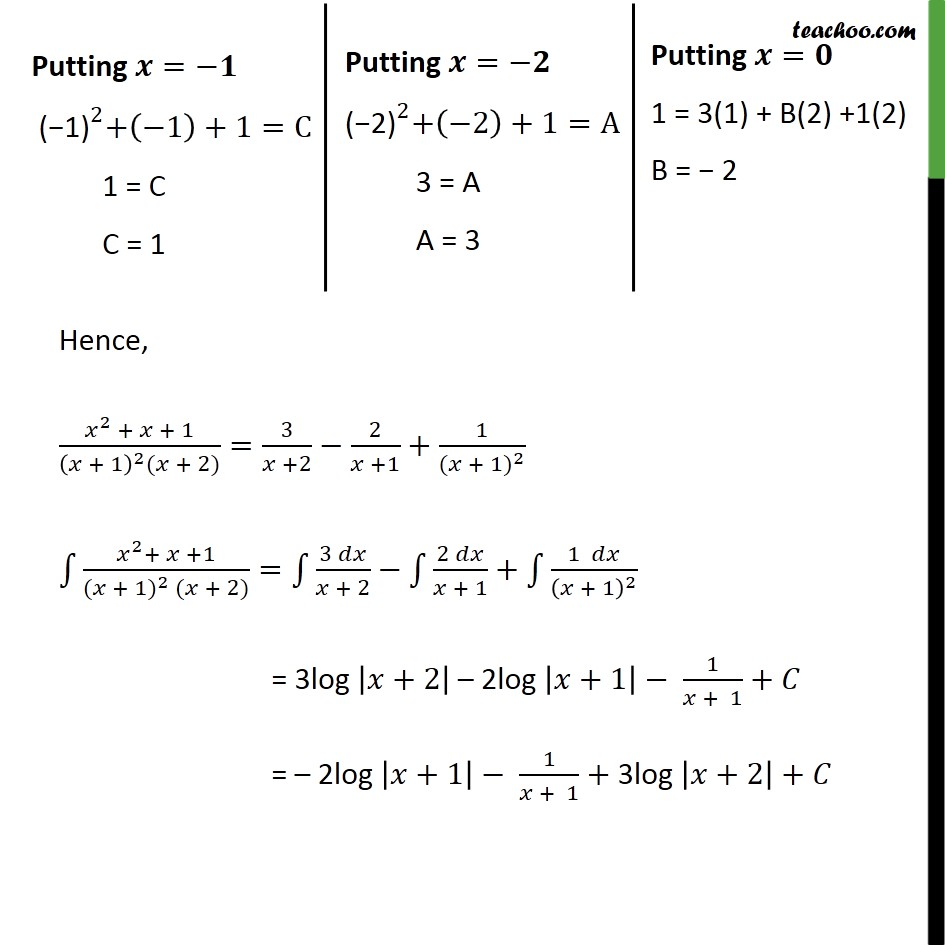1. Chapter 7 Class 12 Integrals (Term 2)
2. Concept wise
3. Integration by partial fraction - Type 4

Transcript

Misc 22 Integrate the function 2 + + 1 + 1 2 + 2 2 + + 1 + 1 2 + 2 By partial fraction 2 + + 1 + 1 2 + 2 = A + 2 + B + 1 + C ( + 1) 2 2 + + 1 + 1 2 + 2 = A ( + 1) 2 + B + 1 ( + 2)+ C( + 2) ( + 1) 2 + 2 Cancelling denominators 2 + +1=A ( +1) 2 +B +2 +1 +C( +2) Hence, 2 + + 1 + 1 2 ( + 2) = 3 +2 2 +1 + 1 ( + 1) 2 2 + +1 ( + 1) 2 ( + 2) = 3 + 2 2 + 1 + 1 + 1 2 = 3log +2 2log +1 1 + 1 + = 2log +1 1 + 1 +3log +2 +